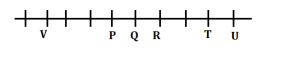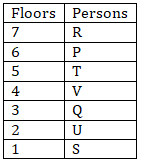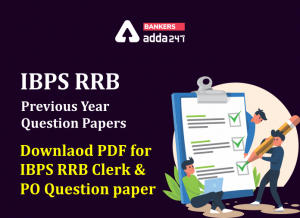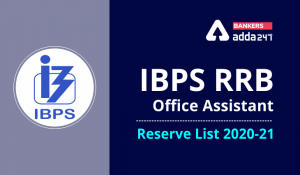Latest Banking jobs   »   Reasoning Ability Quiz For IBPS RRB...

# Reasoning Ability Quiz For IBPS RRB PO, Clerk Prelims 2021- 26th May

Directions (1-5): Study the following information carefully and answer the questions given below:
A certain number of persons are sitting in a linear row and facing towards North direction. T sits second from one of the extreme ends of the row. Three persons are sitting between T and P. One person sits between P and R. As many persons sits to the right of T as there are to the left of V. Q sits fourth to the right of V. Two persons are sitting between P and V. V does not sits next to T. Three persons sit between Q and U. V does not sits next to R. Not more than one person sits between P and Q.

Q1. What is the total number of persons are sitting in the row?
(a) Seven
(b) Five
(c) Six
(d) Eight
(e) Ten

Q2. What is the position of P?
(a) Seventh from the left
(b) Ninth from the right
(c) Fourth from the right
(d) Fifth from the left
(e) Both (b) and (c)

Q3. How many persons are sitting between V and T?
(a) One
(b) Five
(c) Six
(d) Eight
(e) Ten

Q4. Who among the following sits to the immediate right of T?
(a) P
(b) R
(c) Q
(d) U
(e) None of these

Q5. Who among the following sits exactly between P and R?
(a) Q
(b) V
(c) T
(d) U
(e) None of these

Direction (6-10): Study the following information carefully and answer the questions given below:
Seven people P, Q, R, S, T, U and V live on separate floors of a 7-floor building but not necessarily in the same order. Ground floor is as 1st floor, just above floor is as 2nd floor and so on until the topmost floor as 7th floor. V does not live on an odd numbered floor. Two floors gap between S and V, who lives above of S. There is one floor gap between R and T. Q lives just above the floor in which U lives. More than three floors gap between R and U. R lives above of the U’s floor. The number of floors above the P’s floor is same as below to the U’s floor.

Q6. How many floors gap between P and Q?
(a) Two
(b) None
(c) Four
(d) Five
(e) None of these

Q7. Who among the following person lives on the 3rd floor?
(a) T
(b) U
(c) Q
(d) V
(e) None of these

Q8. Four of the following five are alike in a certain way and hence they form a group. Which one of the following does not belong to that group?
(a) R
(b) T
(c) S
(d) Q
(e) P

Q9. The number of floors gap between U and V is same as the number of floors gap between Q and ___?
(a) S
(b) V
(c) R
(d) P
(e) U

Q10. If all the persons are living in alphabetical order from top to bottom, then how many persons remain at the same position?
(a) Five
(b) One
(c) None
(d) Three
(e) Four

Direction (11-15): In each of the questions below are given some statements followed by two conclusions. You have to take the given statements to be true even if they seem to be at variance with commonly known facts. Read all the conclusions and then decide which of the given conclusions logically follows from the given statements disregarding commonly known facts.
(a) If only conclusion I follows.
(b) If only conclusion II follows.
(c) If either conclusion I or II follows.
(d) If neither conclusion I nor II follows.
(e) If both conclusions I and II follow.

Q11. Statements: Q>E=R; R<T≥Y; Y<U
Conclusion I: Q>T II: U≤E

Q12. Statements: A<S; S=F>D; D≤G
Conclusion I: A=F II: S>D

Q13. Statements: F>G=H; H<J≤M; M>N≥V
Conclusion I: F>M II: G≤M

Q14. Statements: X>V; V=R≥E; E≤Q>O
Conclusion I: X>E II: V=Q

Q15. Statements: Z=C>E; E<T≤U; U>P=G
Conclusion I: Z≤T II: U>E

Practice More Questions of Reasoning for Competitive Exams:

###### Study Plan for IBPS RRB PO/Clerk Prelims 2021

Solutions

Solutions (1-5):
Sol.S1. Ans. (e)
S2. Ans. (d)
S3. Ans. (c)
S4. Ans. (d)
S5. Ans. (a)

Solutions (6-10):
Sol.S6. Ans. (a)
S7. Ans. (c)
S8. Ans. (e)
S9. Ans. (a)
S10. Ans. (b)

Solutions (11-15):
S11. Ans. (d)
Sol. I: Q>T (False) II: U≤E (False)

S12. Ans. (b)
Sol. I: A=F (False) II: S>D (True)

S13. Ans. (d)
Sol. I: F>M (False) II: G≤M (False)

S14. Ans. (a)
Sol. I: X>E (True) II: V=Q (False)

S15. Ans. (b)
Sol. I: Z≤T (False) II: U>E (True)#### Congratulations!Incorrect details? Fill the form again here

•IBPS RRB Previous Year Question Paper PD...
•IBPS RRB Clerk 2nd Reserve List 2022 Out...
•Reasoning Ability Quiz For IBPS RRB PO C...
•Reasoning Ability Quiz For IBPS Clerk Pr...
•Reasoning Ability Quiz For IBPS RRB PO P...
•Quantitative Aptitude Quiz For IBPS RRB ...